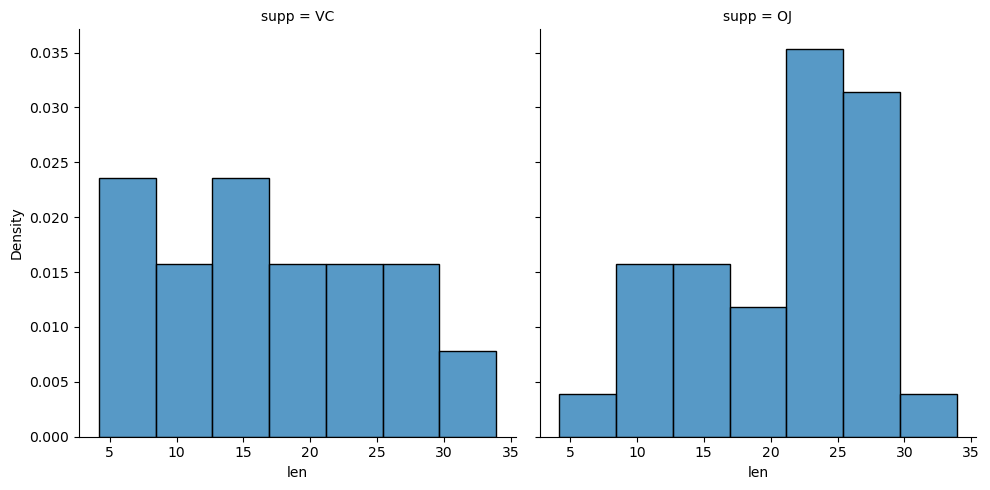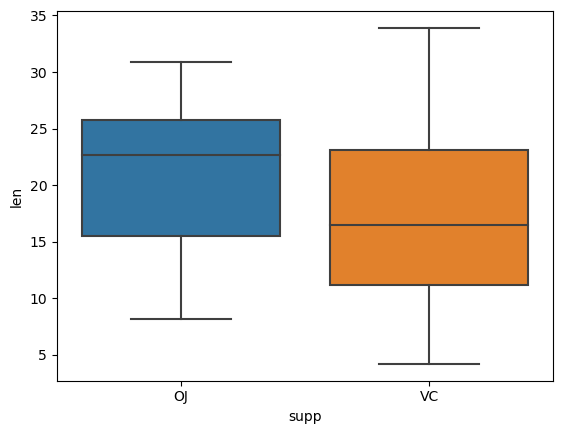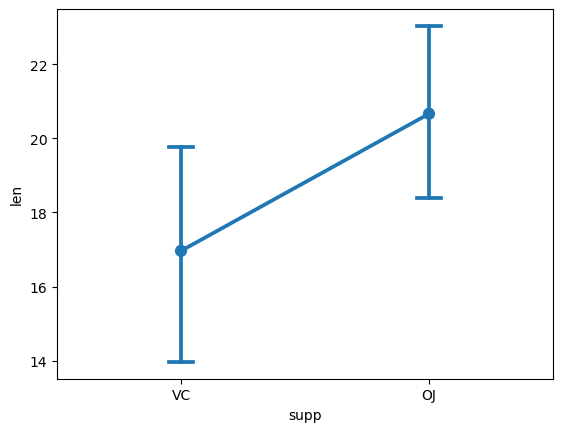# How to create bivariate plots to compare groups (in Python, using Matplotlib and Seaborn)

See all solutions.

Suppose we have a dataset with different treatment conditions and an outcome variable, and we want to perform exploratory data analysis. How would we visually compare the treatment conditions with regards to the outcome variable?

## Solution

The solution below uses an example dataset about the teeth of 10 guinea pigs at three Vitamin C dosage levels (in mg) with two delivery methods (orange juice vs. ascorbic acid). (See how to quickly load some sample data.)

1
2
from rdatasets import data
df = data('ToothGrowth')


If you wish to understand the distribution of a numeric variable (here “len”) compared across different values of a categorical variable (here “supp”), you can construct a bivariate histogram. We use Seaborn and Matplotlib to do so.

1
2
3
4
import seaborn as sns
import matplotlib.pyplot as plt
sns.displot(df, x="len", col="supp", stat="density")
plt.show()To visualize the same information summarized using quartiles only, you can construct a bivariate box plot.

1
2
sns.boxplot(x="supp", y="len", data = df, order = ['OJ','VC'])
plt.show()Even more simply, we may wish to plot just the means and 95% confidence intervals around the mean for the quantitative variable, for each of the values of the categorical variable. We do so with a point plot.

1
2
3
4
sns.pointplot(x = 'supp', y = 'len', data = df,
ci = 95,        # Which confidence interval?  Here 95%.
capsize = 0.1)  # Size of "cap" drawn on each confidence interval.
plt.show()

1
2
3
4
5
/tmp/ipykernel_6175/1597037981.py:1: FutureWarning:

The ci parameter is deprecated. Use errorbar=('ci', 95) for the same effect.

sns.pointplot(x = 'supp', y = 'len', data = df,